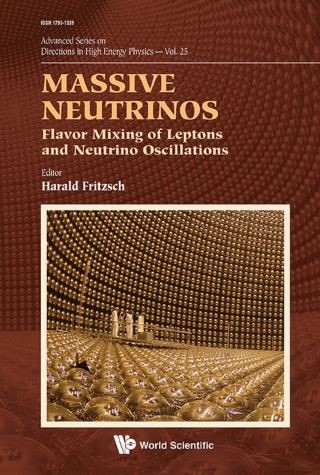## Massive Neutrinos: Flavor Mixing Of Leptons And Neutrino Oscillations• Description
• Author
• Info
• Reviews

### Description

Since the discovery of neutrino oscillations neutrino physics has become an interesting field of research in physics. They imply that neutrino must have a small mass and that the neutrinos, coupled to the charged leptons, are mixtures of the mass eigenstates, analogous to the flavor mixing of the quarks. The mixing angles for the quarks are small, but for the leptons two of the mixing angles are large. The masses of the three neutrinos must be very small, less than 1 eV, but from the oscillation experiments we only know the mass differences — the absolute masses are still unknown. Also we do not know, if the masses of the neutrinos are Dirac masses, as the masses of the charged leptons and of the quarks, or whether they are Majorana masses.

In this volume, an overview of the present state of research in neutrino physics is given by well-known experimentalists and theorists. The contents — originated from talks and discussions at a recent conference addressing some of the most pressing open questions in neutrino physics — range from the oscillation experiments to CP-violation for leptons, to texture zero mass matrices and to the role of neutrinos in astrophysics and cosmology.

Since the discovery of neutrino oscillations neutrino physics has become an interesting field of research in physics. They imply that neutrino must have a small mass and that the neutrinos, coupled to the charged leptons, are mixtures of the mass eigenstates, analogous to the flavor mixing of the quarks. The mixing angles for the quarks are small, but for the leptons two of the mixing angles are large. The masses of the three neutrinos must be very small, less than 1 eV, but from the oscillation experiments we only know the mass differences — the absolute masses are still unknown. Also we do not know, if the masses of the neutrinos are Dirac masses, as the masses of the charged leptons and of the quarks, or whether they are Majorana masses.

In this volume, an overview of the present state of research in neutrino physics is given by well-known experimentalists and theorists. The contents — originated from talks and discussions at a recent conference addressing some of the most pressing open questions in neutrino physics — range from the oscillation experiments to CP-violation for leptons, to texture zero mass matrices and to the role of neutrinos in astrophysics and cosmology.